# Formula Column

Zoho Advanced Analytics app allows you to define your own powerful formulas to meet your specific reporting requirements. This enables you to easily perform simple calculations such as addition and subtraction to a complex combination of in-built functions. As the output of the formula adds a new column in the table is called a Formula Column. You can use these formula columns in creating reports in the same way you use other columns in a table.

## Creating Formula Column

Zoho Advanced Analytics app provides you with a variety of in-built functions, which are predefined mathematical formulas that are designed to perform specific well-known calculations easily. These in-built functions can be used in combination with the table columns and basic arithmetic operators like +, -, / and * to create your formula column.

To create and use formula columns:

1. Open the table in which you want to add the formula column.
2. Select Add > Formula Column option from the toolbar.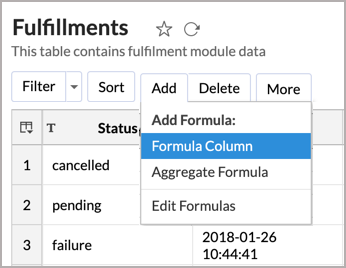Alternatively, you can also right-click on a column in the table and select  Add Formula > Formula Column.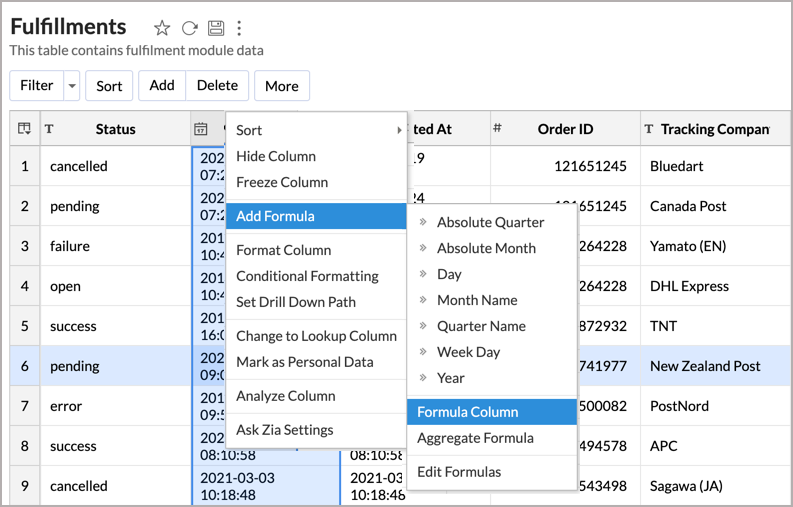3. The Formula Column dialog box will open as shown below. This dialog box provides easy access to the names of the columns in your table, as well as to many of the in-built functions that are available to create formulas. A brief description of the function with syntax and an example will be displayed when you hover your mouse over the information icon inline to a function.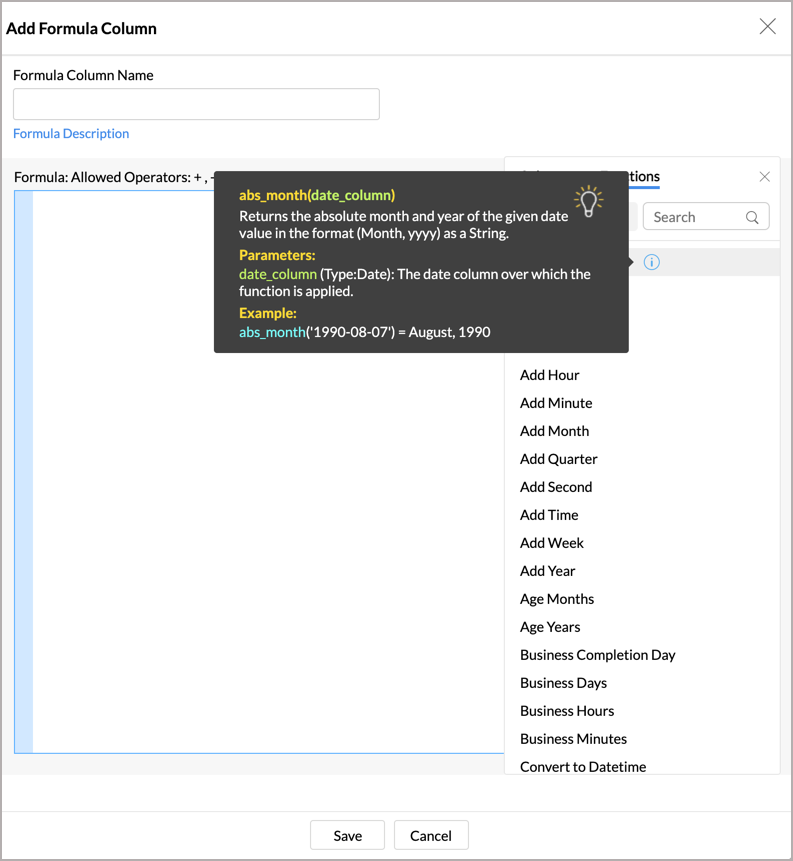4. In the Add Formula Column dialog box, select the columns and functions that you want to insert from the list under the Columns and the Function section.

For example, to build a formula column that calculates the total time of shipping a product in hours,

1. Use the If and Date & Time Diff function under the Functions section. You will notice that the syntax of respective functions appear in the Formula text box.
2. In the Formula text box, position the cursor in between the parenthesis, select the required columns, from the Columns sections, to be added in the formula. Here, we will use Shipping Status, Created At, and Updated At columns.
3. The status of Shipping must be delivered in order to calculate the total shipping time in hours. We will provide this condition as well here.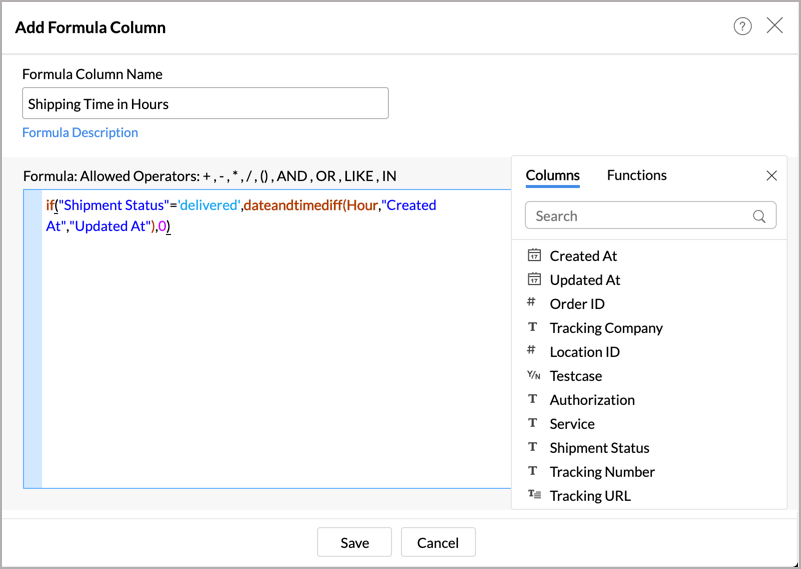4. After adding the formula in the Formula Column Name text box, click Save. A new formula column will be created in your table as shown below.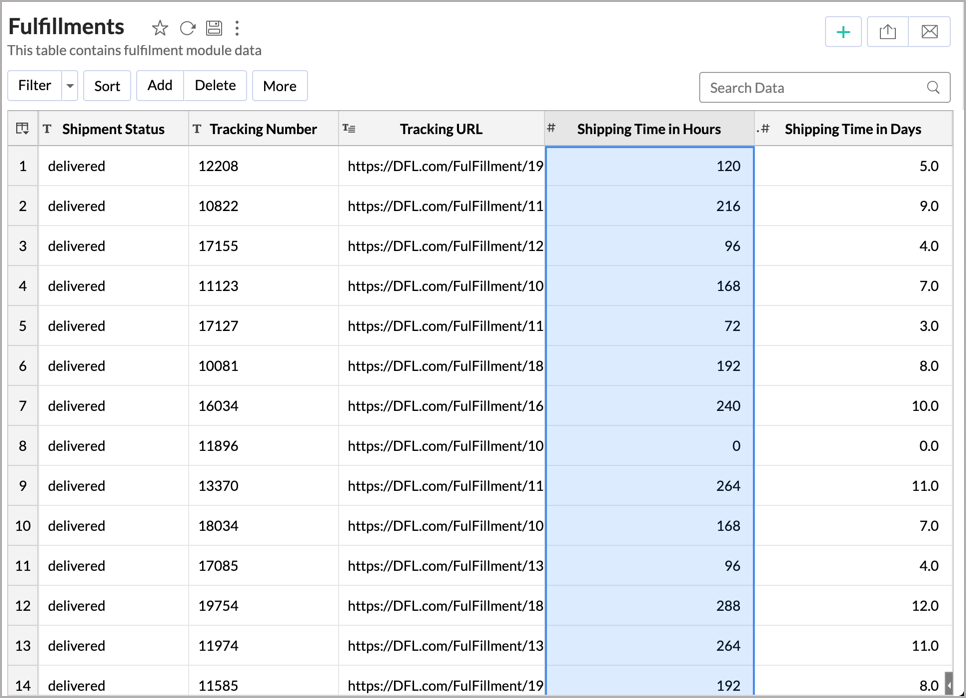You can edit the formula column any time by right-clicking on the formula column listed in the table and clicking Edit Formula Column in the popup menu. Also, refer to View/Edit Formulas to know how you could view all the formulas defined on a table and handle edition or deletion of the same.

## Quick Add Option for Formula Columns

Zoho Advanced Analytics app provides an easy and convenient way to add formula columns that are based on a limited set of widely used functions. You can find this quick add option as follow.

1. Select a column based on which you want to add the formula column.
2. Click Add > Add Formula option on the toolbar. Alternatively, you can also right-click on the required column and click Add Formula from the drop down menu.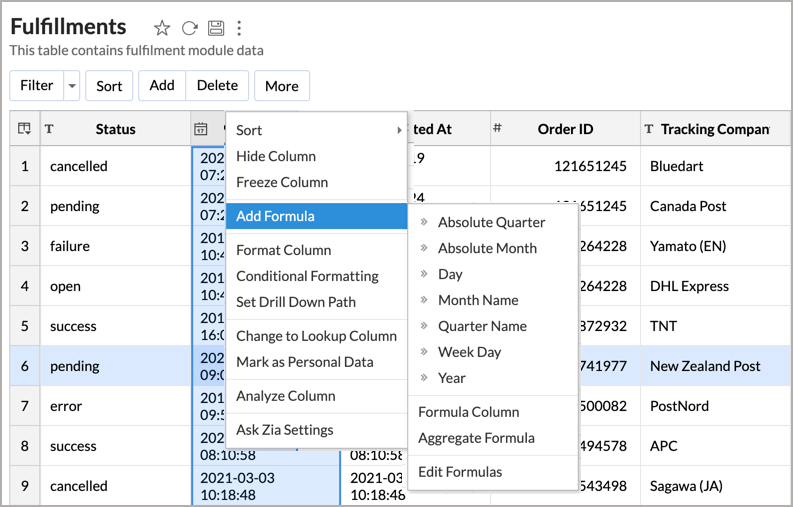3. Zoho Advanced Analytics app automatically identifies the data type of the column selected and displays a list of widely used pre-defined formulas which are applicable. On selecting the required formula from the list, the Zoho Advanced Analytics app applies the formula on the corresponding column and adds a new formula column, at the right end of the table.​

## In-built functions provided by Zoho Advanced Analytics app

Zoho Advanced Analytics app provides you with a variety of in-built functions which are predefined mathematical formulas designed to perform specific well-known calculations easily. Refer to the In-Built Function page to view the complete list of functions.

## Creating Formulas Over Formula Column

Formula columns are similar to other columns of the table. You can make use of existing formula columns while creating a new formula column, as you do with other columns of the table. Reusing existing formulas column to create new formula columns helps you create powerful formula combinations, as well as eases in the maintenance of this formula structure in your Workspace. Refer to the above section to know how to create a formula column.

## Creating Reports Using Formula Column

Formula columns can be used while creating reports as you would use a column in a table. When you create a new report over the table, in which you have added the formula column, all these formula columns will be listed in the Columns list pane on the left, which can be drag and drop to create the report. You can apply functions on the formula column, as you do for other columns, using the combo box that is displayed over the column when dropped in the design area of the report.

The following screenshot shows the Shipping Time in Hours formula column listed in the columns list page.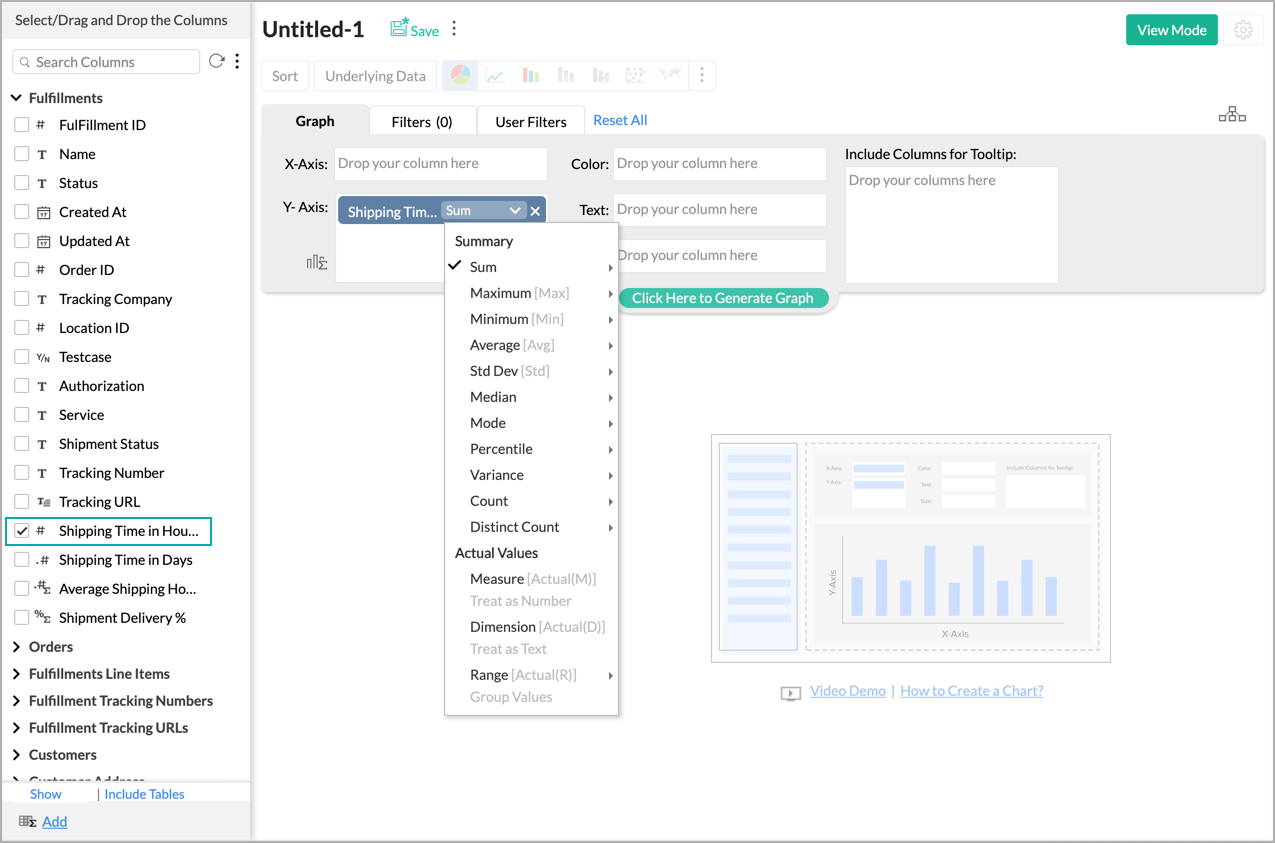## View/Edit Formulas

Zoho Advanced Analytics app allows you to view, edit, format, and delete all the formulas defined for a table through the Edit Formula option. You can view & edit both Formula Columns and Aggregate Formulas using this option. To view/edit existing Formula Column or Aggregate Formulas:

1. Fromo the required table, click Add > Edit Formulas option from the toolbar.
2. The Edit Formula dialog box with a list of all the formulas associated to the table will open as shown below.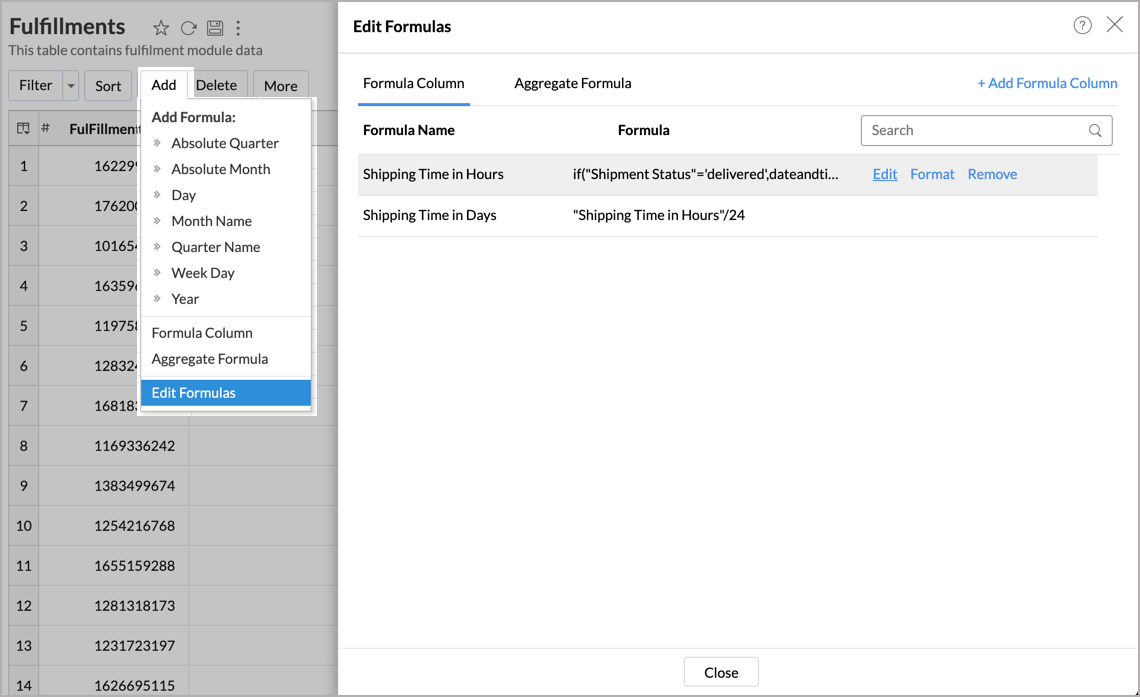3. Click the Edit link next to the formula that you wish to edit.
4. The Edit Formula dialog box will open, you can modify the existing formula the same way as you would create a new formula.
• Edit Formula: You can edit the constructed formula using the Edit link provided next to the corresponding formula.
• Delete Formula: You can delete an existing Formula any time from the table using the Remove link provided next to the corresponding formula.
• Format Formula: You can change the format of the Formula result, like alignment, decimal places, date format, currency symbols, etc., depending on the data type of the formula using the Format link. It's similar to how you format a data column.